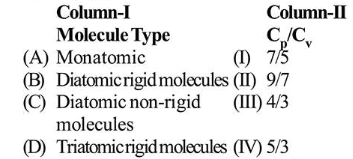# Match the Cp/Cv ratio for ideal gases with different type of molecules:Question:

Match the $\mathrm{C}_{\mathrm{p}} / \mathrm{C}_{\mathrm{v}}$ ratio for ideal gases with different type of molecules:1. (1) (A)-(IV), (B)-(II), (C)-(I), (D)-(III)

2. (2) (A)-(III), (B)-(IV), (C)-(II), (D)-(I)

3. (3) (A)-(IV), (B)-(I), (C)-(II), (D)-(III)

4. (4) (A)-(II), (B)-(III), (C)-(I), (D)-(IV)

Correct Option: , 3

Solution:

(3) As we know,

$\gamma=\frac{C_{p}}{C_{v}}=1+\frac{2}{f}$, where $f=$ degree of freedom

(A) Monatomic, $f=3$

$\therefore \gamma=1+\frac{2}{3}=\frac{5}{3}$

(B) Diatomic rigid molecules, $f=5$

$\therefore \gamma=1+\frac{2}{5}=\frac{7}{5}$

(C) Diatomic non-rigid molecules, $f=7$

$\therefore \gamma=1+\frac{2}{7}=\frac{9}{7}$

(D) Triatomic rigid molecules, $f=6$

$\therefore \gamma=1+\frac{2}{6}=\frac{4}{3}$